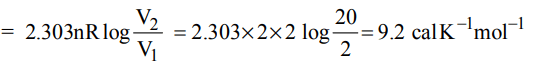## Thermodynamics Questions and Answers Part-3

1. Hess’s law deals with :
a) changes in heats of reaction
b) rates of reactions
c) equilibrium constants
d) influence of pressure on the volume of a gas

Explanation: Hess's law deals with changes in heat of reaction

2. If a chemical change is brought about by one or more methods in one or more steps, then the amount of heat absorbed or evolved during the complete change is the same whichever method was followed. This rule is known as :
a) Le Chatelier principle
b) Hess’s law
c) Joule Thomson effect
d) None of these

Explanation: Hess's law

3. One mole of an ideal gas at 300 K is expanded isothermally from an initial volume of 1 litre to 10 litres. The DE for this process is $\left(R = 2 cal mol^{-1}K^{-1}\right)$
a) 163.7 cal
b) zero
c) 1381.1 cal
d) 9 lit atm

Explanation: For a finite change $\triangle$E = Cv$\triangle$T . For an isothermal process $\triangle$T = 0. Hence $\triangle$E = 0

4. One mole of an ideal gas is allowed to expand reversibly and adiabatically from a temperature of 27ºC. If the work done during the process is 3 kJ, the final temperature will be equal to $\left(C_{V} = 20 JK^{-1}\right)$
a) 150 K
b) 100 K
c) 26.85 K
d) 295 K

Explanation: Work done during adiabatic expansion = CV (T2 - T1 )
-3000 = 20(T2 -300)
T2 = 150K

5. 2 moles of an ideal gas at 27ºC temperature is expanded reversibly from 2 lit to 20 lit. Find entropy change $\left(R = 2 cal/mol K\right)$
a) 92.1
b) 0
c) 4
d) 9.2

Explanation: Entropy change at constant temperature6. The heat required to raise the temperature of a body by 1K is called
a) specific heat
b) thermal capacity
c) water equivalent
d) none of these

Explanation: Concept of thermal capacity is, the heat required to raise the temperature of a body

7. For a reaction to occur spontaneously
a) $\triangle S$   must be negative
b) $\left(\triangle H-T\triangle S\right)$
c) $\left(\triangle H+T\triangle S\right)$
d) $\triangle H$   must be negative

Explanation: $\triangle$G= $\triangle$H-T$\triangle$S , $\triangle$G must be negative hence $\triangle$H -T$\triangle$S must be negative

8. The enthalpy and entropy change for a chemical reaction are $– 2.5\times10^{3}cal$     and 7.4 cal $deg^{-1}$   respectively. The reaction at 298K will be
a) spontaneous
b) reversible
c) irreversible
d) non – spontaneous

Explanation:9. Heat exchanged in a chemical reaction at constant temperature and constant pressure is called
a) internal energy
b) enthalpy
c) entropy
d) free energy

Explanation: qp = $\triangle$H (Enthalpy)
10. For the process Dry ice $\rightarrow CO_{2}\left(g\right)$
a) $\triangle H$   is positive and $\triangle S$   is negative
b) Both $\triangle H$   and $\triangle S$  are negative
c) Both $\triangle H$   and $\triangle S$   are positive
d) $\triangle H$   is negative whereas $\triangle S$   is positive
Explanation: Endothermic process, increase in entropy, $\triangle$H = +ve, $\triangle$S =+ ve